## Info

Publication number
JP5896795B2
JP5896795B2 JP2012057811A JP2012057811A JP5896795B2 JP 5896795 B2 JP5896795 B2 JP 5896795B2 JP 2012057811 A JP2012057811 A JP 2012057811A JP 2012057811 A JP2012057811 A JP 2012057811A JP 5896795 B2 JP5896795 B2 JP 5896795B2
Authority
JP
Japan
Prior art keywords
unit
correlation
value
plurality
threshold
Prior art date
Legal status (The legal status is an assumption and is not a legal conclusion. Google has not performed a legal analysis and makes no representation as to the accuracy of the status listed.)
Expired - Fee Related
Application number
JP2012057811A
Other languages
Japanese (ja)
Other versions
JP2013192107A5 (en
JP2013192107A (en
Inventor

Original Assignee

Priority date (The priority date is an assumption and is not a legal conclusion. Google has not performed a legal analysis and makes no representation as to the accuracy of the date listed.)
Filing date
Publication date
Application filed by 三菱電機株式会社 filed Critical 三菱電機株式会社
Priority to JP2012057811A priority Critical patent/JP5896795B2/en
Publication of JP2013192107A publication Critical patent/JP2013192107A/en
Publication of JP2013192107A5 publication Critical patent/JP2013192107A5/ja
Application granted granted Critical
Publication of JP5896795B2 publication Critical patent/JP5896795B2/en
Application status is Expired - Fee Related legal-status Critical
Anticipated expiration legal-status Critical

## Images

•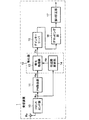•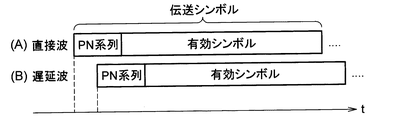•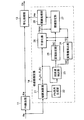•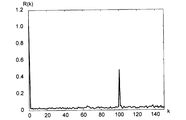•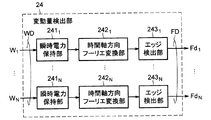•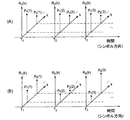•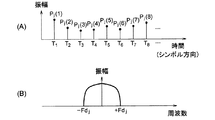•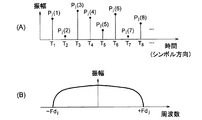•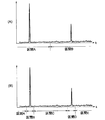•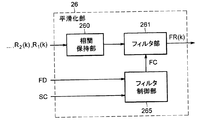•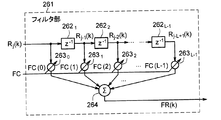•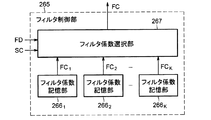•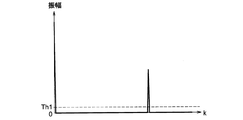•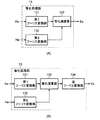•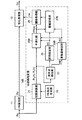•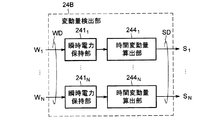••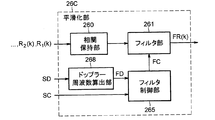## Description

The present invention relates to an equalization technique for compensating for distortion received by a received signal from a transmission path, and more particularly, to an equalization technique for compensating for distortion received by a received signal from a transmission path using a known signal sequence.

In wireless communication systems and digital terrestrial broadcasting systems, a receiver not only receives an incoming wave (direct wave) that directly arrives from a transmitter, but also reflects, diffracts, or is reflected by an obstacle such as a building after being transmitted from the transmitter. Incoming waves (indirect waves) that have been scattered may also be received. The delay profile indicates information regarding the delay time, amplitude, and phase of such a plurality of incoming waves. Japanese Patent Laying-Open No. 2011-199391 (Patent Document 1) discloses a transmission path response estimator that estimates a transmission path response using a delay profile estimation result.

The transmission path response estimator disclosed in Patent Document 1 calculates a time correlation between a pseudo-random sequence (PN sequence: Pseudo-random Noise series) included in a received signal and a known pseudo-random sequence generated by itself. A correlation unit that generates a correlation waveform, a smoothing unit that smoothes the correlation waveform in the time (symbol) direction, and an effective estimation of the delay profile by selecting only the peak portion of the output of the smoothing unit. And a path determination unit. Since the smoothing unit smoothes the correlation waveform in the time (symbol) direction, it is possible to suppress the noise component included in the delay profile estimation result.

Japanese Patent Laying-Open No. 2011-199391 (paragraphs 0051 to 0057, FIGS. 7 and 11, etc.)

However, the technique disclosed in Patent Document 1 has a problem that the estimation accuracy of the delay profile is lowered in a reception environment where the receiver moves relative to the transmitter.

In view of the above, an object of the present invention is to provide an equalization apparatus, a reception apparatus, and an equalization method that can suppress a decrease in delay profile estimation accuracy even in an environment in which the receiver moves.

The equivalent apparatus according to the first aspect of the present invention provides a transmission symbol received by a receiving apparatus when a transmission symbol having a known signal sequence and an effective symbol including an information signal is continuously transmitted from the transmitting apparatus. An equalization apparatus for correcting distortion of a received signal, wherein a known signal sequence generation unit that supplies a known signal sequence and calculates a cross-correlation between the known signal sequence and the received signal for each transmission symbol A correlation calculation unit that obtains a sequence of cross-correlation values, and detects positions of a plurality of correlation peak values respectively corresponding to a plurality of incoming waves received by the receiving apparatus from the cross-correlation value sequence for each transmission symbol. an arrival wave detection unit, based on the respective positions of the cross-correlation value with respect to sequence of the cross-correlation values, including a section corresponding to at least one of the plurality of correlation peak values, a plurality A section setting unit for setting a segment, the changing amount detecting unit for detecting a time variation of the plurality of correlation peak value, the cross-correlation value of a sequence symbol direction smoothing filtering each section of the plurality of sections with respect to And a filter control for changing the smoothing filtering characteristic of the filter unit for each of the sections according to the time variation detected by the variation amount detection unit. And an equalization processing unit that executes an equalization process for correcting distortion of the received signal based on the estimated delay profile, and the filter control unit passes through the filter unit as the time variation increases. The smoothing filtering characteristic is changed so as to widen the band.

A receiving apparatus according to a second aspect of the present invention is a signal receiving unit that receives a signal of a transmission symbol when transmission symbols having a known signal sequence and an effective symbol including an information signal are continuously transmitted from the transmission apparatus. And an equalizing device according to a first aspect for correcting distortion of the received signal received by the signal receiving unit.

The equivalent method according to the third aspect of the present invention is such that when a transmission symbol having a known signal sequence and an effective symbol including an information signal is continuously transmitted from a transmission device, the transmission symbol received by the reception device is transmitted. An equalization method for correcting distortion of a received signal, calculating a cross-correlation between the known signal sequence and the received signal for each transmission symbol to obtain a cross-correlation value sequence, and the cross-correlation From the sequence of values, detecting a position of a plurality of correlation peak values respectively corresponding to a plurality of incoming waves received by the receiving device for each transmission symbol, and based on each position of the cross-correlation value, relative sequence of the cross-correlation values, said comprising a plurality of sections corresponding to at least one of the correlation peak value, and setting a plurality of sections, said plurality of correlation peak values Detecting a time variation, and generating an estimated delay profile smoothing filtering symbol direction with respect to sequence of the cross-correlation value by performing for each section of the plurality of sections, the detected time variation And changing the characteristics of the smoothing filtering for each of the plurality of sections, and correcting distortion of the received signal based on the estimated delay profile. The step of changing is characterized in that the characteristic of the smoothing filtering is changed so that the pass band in the smoothing filtering becomes wider as the time variation is larger.

According to the present invention, the filter control unit changes the filter characteristic in accordance with the temporal fluctuation of the correlation peak value detected by the fluctuation amount detection unit. Therefore, even in an environment where the transmission path characteristic changes with time, the delay profile A decrease in estimation accuracy can be suppressed. For this reason, it is possible to suppress a decrease in delay profile estimation accuracy even in an environment where the receiver moves.

It is a functional block diagram which shows roughly the structural example of the receiver of Embodiment 1 which concerns on this invention. (A), (B) is a figure which shows roughly the example of several incoming waves (a direct wave and a delay wave). 3 is a functional block diagram schematically showing a configuration of a transmission path estimation unit according to Embodiment 1. FIG. It is a graph which shows an example of the correlation series with respect to a 2 wave model transmission path. 3 is a functional block diagram schematically showing a configuration of a variation amount detection unit of the first embodiment. FIG. (A) and (B) are diagrams schematically illustrating a correlation sequence (delay profile). (A) is a diagram schematically showing an example of a sequence of correlation peak values in the symbol direction, and (B) is a diagram schematically showing a frequency spectrum corresponding to the sequence of correlation peak values in (A). is there. (A) is a figure which shows roughly the other example of the series of the correlation peak value in a symbol direction, (B) shows the frequency spectrum corresponding to the series of the correlation peak value of (A) roughly. FIG. (A), (B) is a figure which shows the example of the area set by the area setting part of Embodiment 1. FIG. FIG. 3 is a diagram schematically illustrating a configuration example of a smoothing unit according to the first embodiment. FIG. 3 is a diagram schematically illustrating a configuration example of a filter unit according to the first embodiment. 3 is a functional block diagram schematically showing a configuration example of a filter control unit according to Embodiment 1. FIG. It is a graph which shows roughly an example of the output of the threshold value process part with respect to a 2 wave model transmission path. (A) is a figure which shows roughly the structure of the equalization process part corresponding to a multicarrier modulation system, (B) shows the structure of the equalization process part corresponding to a single carrier modulation system roughly FIG. It is a functional block diagram which shows roughly the structure of the transmission path estimation part of Embodiment 2 which concerns on this invention. FIG. 6 is a functional block diagram schematically showing a configuration of a variation amount detection unit of a second embodiment. FIG. 10 is a functional block diagram schematically showing a configuration example of a smoothing unit in a second embodiment. It is a functional block diagram which shows schematically the structure of the smoothing part of Embodiment 3 which concerns on this invention.

Hereinafter, various embodiments according to the present invention will be described with reference to the drawings.

Embodiment 1 FIG.
FIG. 1 is a functional block diagram schematically showing a configuration example of the receiving device 1 according to the first embodiment of the present invention. As shown in FIG. 1, the receiving apparatus 1 includes a receiving antenna element Rx, a front end unit 10, a PN removing unit 11, an equalizing unit 12, a deinterleaver 15, a demapping unit 16, and an error correcting unit 17. ing.

The front end unit 10 receives a radio signal via the receiving antenna element Rx. The front end unit 10 performs analog signal processing such as tuning processing on the radio signal to generate a carrier band signal, and further performs A / D conversion and orthogonal demodulation on the carrier band signal to generate a baseband received signal ( Complex digital signal) Rs is generated and output.

2A and 2B are diagrams schematically illustrating an example of a plurality of incoming waves (direct wave and delayed wave) received by the receiving apparatus 1. FIG. As shown in FIG. 2, the incoming wave is transmitted with a transmission symbol composed of a PN sequence as a known signal sequence and an effective symbol including an information signal as one unit. Here, a PN (Pseudo-Random Noise) sequence is also called a pseudo-random sequence, and is composed of several hundred symbols (time domain signals) that are continuous. On the other hand, the effective symbol is a signal group generated by a single carrier modulation method that transmits information using a single carrier wave, or a multi-carrier modulation method that transmits information using a plurality of carrier waves (subcarriers) (particularly, (Orthogonal frequency division multiplexing system). Such a transmission symbol format is adopted in, for example, the DTMB (Digital Terrestrial Multimedia Broadcasting) standard which is a digital terrestrial broadcasting standard in China.

The PN removing unit 11 removes the PN sequence from the baseband received signal Rs sequence, and equalizes a time domain signal Re (hereinafter referred to as an effective symbol signal Re) of only effective symbols in the baseband received signal Rs. 12 is output.

The equalization unit 12 uses the channel estimation unit 14 that estimates channel characteristics (channel impulse response) from the baseband received signal Rs sequence, and the channel characteristic estimation value He supplied from the channel estimation unit 14. And an equalization processing unit 13 for performing frequency axis equalization (equalization in the frequency domain) on the effective symbol signal Re.

As shown in FIGS. 2 (A) and 2 (B), the receiving device 1 is directly reflected from a transmitting device (not shown) and reflected, diffracted or scattered by an obstacle such as a building. There are cases where a delayed wave that arrives after being delayed with respect to the direct wave is received. In this case, the receiving apparatus 1 receives a signal in which a direct wave transmission symbol and a delayed wave transmission symbol are superimposed. Although only one delayed wave is shown in FIG. 2B, the present invention is not limited to this. The receiving device 1 may receive a signal in which two or more delayed waves and a direct wave are superimposed. The equalization unit 12 has a function of appropriately correcting distortion (phase rotation amount and amplitude change) of the baseband reception signal Rs due to the influence of the delayed wave.

Referring to FIG. 1, the deinterleaver 15 performs a deinterleave process on the equalized signal Es output from the equalization processing unit 13. The demapping unit 16 performs demapping processing on the output of the deinterleaver 15. Specifically, the demapping unit 16 converts the output (data symbol) of the deinterleaver 15 into a bit string in accordance with the carrier modulation scheme used in the transmission apparatus. Examples of carrier modulation schemes include, but are not limited to, digital modulation schemes such as QPSK (Quadrature Phase Shift Keying) and 2 M- value QAM (Quadrature Amplitude Modulation; M is a positive integer). The error correction unit 17 performs, for example, known Reed-Solomon decoding or convolutional decoding on the output sequence of the demapping unit 16 to generate and output a data signal sequence.

FIG. 3 is a functional block diagram schematically showing the configuration of the transmission path estimation unit 14 of the first embodiment. As illustrated in FIG. 3, the transmission path estimation unit 14 includes a correlation calculation unit 21, a PN sequence generation unit 22, a smoothing unit 26, and a threshold processing unit 28. The transmission path estimation unit 14 further includes an incoming wave detection unit 23, a fluctuation amount detection unit 24, a section setting unit 25, and a threshold setting unit 27.

When the received PN sequence is input as the baseband received signal Rs sequence, the PN sequence generating unit 22 self-generates a PN sequence corresponding to the received PN sequence and supplies the PN sequence to the correlation calculating unit 21. Correlation calculation unit 21 calculates a cross-correlation between baseband received signal Rs and the PN sequence for each transmission symbol to obtain a cross-correlation value sequence (hereinafter also referred to as “correlation sequence”). The cross-correlation can be expressed as a function related to a shift time (delay time) τ when one signal waveform of the two signal waveforms is delayed by the time τ with respect to the other signal waveform.

Assume a case of an AWGN (Additive white Gaussian noise) transmission line in which only one incoming wave to the receiving apparatus 1 exists. When the sample number corresponding to the delay time τ is an integer k, the transmission symbol number is an integer j, and the number of samples is an integer L, the correlation sequence R j (k) between the baseband received signal Rs and the PN sequence is It can be expressed by equation (1).

Here, pn (ik) represents a transmission PN sequence, f j (ik) represents a valid data signal in a valid symbol in the jth transmission symbol, and n j (ik) represents It represents Gaussian noise received by the jth transmission symbol, and pn (i) represents a self-generated PN sequence.

By arranging the above equation (1), the following equation (2) can be obtained.

Here, δ (k) in the first term on the right side of the equation (2) is defined by the following equation (3).

Δ (k) in the first term on the right side of the above equation (2) has a very sharp correlation peak value when k = 0 corresponding to the time when the incoming wave arrives at the receiving apparatus 1, and other than k = 0 Is a function having a value almost close to zero.

When there are a plurality of arriving waves, the receiving apparatus 1 receives a signal in which the plurality of arriving waves are superimposed on each other, so that a plurality of sharp peaks appear in the correlation sequence at a plurality of times corresponding to the plurality of arriving waves. In the range corresponding to other times, a noise component as shown in the second term on the right side of the above equation (2) appears in the correlation sequence. FIG. 4 is a graph showing an example of the correlation sequence R (k) for the two-wave model transmission path. In this two-wave model transmission line, the DU ratio (Desired to Unsigned signal ratio) is 6 dB, and the number of samples related to the delay time τ is 100 samples. In FIG. 4, there are peaks indicating the reception levels of the main wave and the delayed wave at k = 0 and k = 100, respectively, and the noise component of the second term of the above equation (1) is present in the other ranges.

Since the transmission apparatus transmits a signal with transmission symbols having the format shown in FIG. 2A as transmission units, the correlation calculation unit 21 of the reception apparatus 1 performs correlation sequence R 1 (k) for each transmission symbol interval. , R 2 (k), R 3 (k),... These correlation sequences R 1 (k), R 2 (k), R 3 (k),... Are given to the smoothing unit 26 and the incoming wave detection unit 23.

The incoming wave detection unit 23 detects the position of the correlation peak value corresponding to each incoming wave for each transmission symbol based on the instantaneous power or amplitude (cross-correlation value) for each sample of the signal output from the correlation calculation unit 21. It has the function to do. Specifically, the incoming wave detection unit 23 calculates the distribution of instantaneous power from the correlation sequence, compares each instantaneous power with a predetermined threshold value, and determines the position of the instantaneous power that is equal to or greater than the threshold value based on the comparison result. The signal WD indicating the instantaneous power at this position is output. In this embodiment, the signal WD indicating the instantaneous power value is output, but a signal indicating the amplitude (correlation peak value) may be output instead.

The fluctuation amount detection unit 24 has a function of detecting temporal fluctuations of the correlation peak value (or its instantaneous power) of each incoming wave based on the signal WD output from the incoming wave detection unit 23. The fluctuation amount detection unit 24 gives the signal FD indicating the detection result to the smoothing unit 26 and the threshold setting unit 27.

FIG. 5 is a functional block diagram schematically showing the configuration of the fluctuation amount detection unit 24 of the first embodiment. As shown in FIG. 5, the fluctuation amount detection unit 24 holds N instantaneous powers W 1 ,..., W 2 respectively corresponding to N incoming waves for a plurality of transmission symbols (N is .., 241 N including an instantaneous power holding unit (signal holding unit) 241 1 ,. For example, FIG. 2 (A), the held in when incoming waves as shown is that there are two, the sequence of the instantaneous power of the direct wave (or amplitude) in the instantaneous power holding portion 241 1 (B), delay instantaneous power series of waves may be employed to hold the instantaneous power holding unit 241 2. Here, the number N of instantaneous power holding portion 241 1 ~241 N, it is necessary for the number of incoming waves is assumed.

Changing amount detecting unit 24 is further instantaneous power holding portion 241 1, ..., N number of frequency spectra by performing a Fourier transform of the time-axis direction with respect to the instantaneous power supplied from each of 241 N (symbol direction) time axis direction Fourier transform unit 242 1 to generate, ..., and 242 N, the frequency value of the N frequency spectrum of the edge (i.e., the maximum Doppler frequency) Fd 1, ..., edge detection unit 243 1 for detecting the Fd N ,..., 243 N are included. Instead of the time axis direction Fourier transform units 242 1 ,..., 242 N , a processing unit that performs orthogonal transform other than Fourier transform may be used.

6A and 6B are diagrams schematically illustrating a correlation sequence (delay profile) in an environment in which the receiving apparatus 1 receives two incoming waves. FIGS. 6A and 6B show correlation sequences generated at times T 1 , T 2 , and T 3 , respectively. The correlation sequences at time T 1 correspond to direct waves and delayed waves, respectively. Correlation peak values P 1 (1), P 2 (1), and the correlation sequence at time T 2 has two correlation peak values P 1 (2), P 2 (2), and time T 3 The correlation sequence has two correlation peak values P 1 (3) and P 2 (3). 6A shows a correlation sequence when the receiving apparatus 1 is stationary with respect to the transmitting apparatus, and FIG. 6B shows a correlation sequence when the receiving apparatus 1 is moving with respect to the receiving apparatus 1. FIG. When the receiving apparatus 1 is stationary, the delay profile characteristics of the correlation series at any time are the same as shown in FIG. On the other hand, at the time of mobile reception, as shown in FIG. 6B, the delay profile characteristics of the correlation sequence change from moment to moment, and the correlation peak values and phases of the direct wave and the delayed wave change with time. .

FIG. 7A is a diagram schematically illustrating a sequence in the time axis direction (symbol direction) of the correlation peak value of the j-th incoming wave, and FIG. 7B is a diagram illustrating the correlation of FIG. It is a figure which shows roughly the frequency spectrum obtained by Fourier-transforming the instantaneous electric power value of peak value Pj (1), Pj (2), .... The frequency spectrum in FIG. 7B is distributed in a frequency range of −Fd j to + Fd j . The frequency value (absolute value) Fd j of the edge of this frequency spectrum is called the maximum Doppler frequency.

On the other hand, FIG. 8A schematically shows a time-axis direction (symbol direction) sequence of the correlation peak value of the j-th incoming wave in a reception environment different from those in FIGS. 7A and 7B. 8B is a frequency spectrum obtained by Fourier transforming the instantaneous power values of the correlation peak values P j (1), P j (2),... Of FIG. FIG. As shown in FIGS. 7A and 8A, the distortion that the incoming wave in the case of FIG. 8A receives from the transmission line is the distortion that the incoming wave in the case of FIG. 7A receives from the transmission line. Greater than distortion. Therefore, the frequency spectrum of FIG. 8B is distributed in a wider range (−Fd j to + Fd j ) in the frequency direction than the frequency spectrum of FIG. 7B, and the maximum of the frequency spectrum of FIG. It has a maximum Doppler frequency greater than the Doppler frequency.

For example, when the arrival direction of the incoming wave is perpendicular to the moving direction of the receiving apparatus 1, the maximum Doppler frequency is approximately 0 Hz. Further, when the arrival direction of the incoming wave is the same as the moving direction of the receiving device 1, the frequency spectrum spreads by the maximum Doppler frequency corresponding to the moving speed. Therefore, as the relative moving speed of the receiving device 1 increases, the distortion that the incoming wave receives from the transmission path increases. For this reason, the maximum Doppler frequency Fd j tends to increase as the relative movement speed increases.

Edge detector 243 1 of FIG. 5, ..., 243 N, the maximum Doppler frequency Fd 1 respectively corresponding to the N incoming waves, ..., it is possible to detect the Fd N. Signals FD indicating these maximum Doppler frequencies Fd 1 ,..., Fd N are given to the smoothing unit 26 and the threshold setting unit 27.

In this embodiment, the fluctuation amount detection unit 24 detects the maximum Doppler frequencies Fd 1 ,..., Fd N based on the instantaneous powers W 1 ,..., W N. It may be configured to detect the Doppler frequency based on the peak value. In the present embodiment, the fluctuation amount detection unit 24 has a configuration in which N instantaneous power sequences respectively corresponding to a maximum of N incoming waves are processed in parallel. It may be configured to detect the maximum Doppler frequencies Fd 1 ,..., Fd N by time-sharing the instantaneous power series. By employing the time division processing, the circuit scale can be reduced.

The section setting unit 25 sets a plurality of sections in the delay time direction for the correlation sequence based on the output of the incoming wave detection unit 23, and the signal SC indicating these sections is smoothed by the threshold unit 26 and the threshold setting unit 27. The function to supply to. Each section may be determined so as to correspond to at least one of the plurality of correlation peaks detected by the incoming wave detection unit 23, or may correspond to each of the plurality of correlation peak values and do not overlap each other. It is also possible to define a plurality of sections. FIG. 9A is a diagram showing sections A and B corresponding to two correlation peaks, respectively. FIG. 9B is a diagram showing sections B and D corresponding to two correlation peaks, and sections A, C and E other than these.

Next, for each section set by the section setting unit 25, the smoothing unit 26 performs symbol direction for a plurality of correlation sequences R 1 (k), R 2 (k), R 3 (k),. A function of executing smoothing filtering to generate an estimated delay profile FR (k); Based on the signal SD indicating the maximum Doppler frequencies Fd 1 ,..., Fd N , the smoothing unit 26 has a function of changing its own smoothing filtering characteristic so as to follow the time variation of the correlation sequence (correlation peak value). Also have.

FIG. 10 is a functional block diagram schematically showing a configuration example of the smoothing unit 26. As shown in FIG. 10, the smoothing unit 26 temporarily stores correlation sequences R 1 (k), R 2 (k), R 3 (k),... For a plurality of transmission symbols. 260, a filter unit 261 that performs smoothing filtering for each section on the plurality of correlation sequences read from the correlation holding unit 260, and a filter control unit 265. The data amount of the correlation sequence held by the correlation holding unit 260 depends on the number of taps of the subsequent filter unit 261. For example, when the subsequent filter unit 261 is an FIR filter having T taps (T is a positive integer), it is only necessary to hold correlation sequences for T symbols.

The filter control unit 265 sets the filter coefficient group FC of the filter unit 261 according to the signals FD and SC so that the filter characteristic (smoothing filtering characteristic) of the filter unit 261 follows the time variation of the section change and the correlation sequence. It has the function to do.

Specifically, the filter control unit 265 can set the variable filter coefficient group FC so that the pass band of the filter unit 261 becomes wider as the maximum Doppler frequency is higher. In the technique described in Patent Document 1, since the smoothing filter coefficient cannot be changed, the filter characteristics cannot follow this change when the maximum Doppler frequency spread changes. .

As the filter unit 261, for example, a known digital filter such as a known FIR (Finite Impulse Response) filter or IIR (Infinite Impulse Response) filter may be used. FIG. 11 is a diagram schematically illustrating a configuration example of the filter unit 261. As illustrated in FIG. 11, the filter unit 261 includes delay elements 262 1 to 262 L−1 , multipliers 263 0 to 263 L−1, and a sum calculator 264. The filter unit 261 includes variable filter coefficients FC (0), FC (1),..., FC (L−1) and input signals R j (k), R j−1 (k) ,. The estimated delay profile FR (k) can be calculated by performing a product-sum operation with k). When a plurality of sections are set by the section setting unit 25, the filter unit 261 executes smoothing filtering for each section by switching the variable filter coefficient group FC for each section.

For example, the filter control unit 265 has a pass band that is twice the maximum Doppler frequency (= Fd j × 2) corresponding to the spread of the frequency spectrum for each section determined by the section setting unit 25. The variable filter coefficient group FC can be set in For example, when sections A and B are set as shown in FIG. 9A, the maximum Doppler frequency of the instantaneous power (or correlation peak value) of the correlation peak value in section A is Fa [Hz. ], A variable filter coefficient group FC having a low-pass filter characteristic in a pass band of 2 × Fa [Hz] is calculated, and the maximum Doppler frequency of the instantaneous power (or correlation peak value) of the correlation peak value in the section B is Fb [Hz. ], It is possible to calculate the variable filter coefficient group FC of the low-pass filter characteristics in the passband of 2 × Fb [Hz].

The filter control unit 265 may calculate the variable filter coefficient group FC, or select a variable filter coefficient group FC corresponding to the signals FD and SC from a plurality of variable filter coefficient groups prepared in advance. Also good. FIG. 12 is a functional block diagram schematically illustrating a configuration example of the filter control unit 265 when the variable filter coefficient group FC is selected from a plurality of variable filter coefficient groups prepared in advance. Filter control unit 265 of FIG. 12, the K filter coefficient group FC 1 ～FC K supplies the K filter coefficient storage unit 266 1, ..., and 266 K, the signal FC, according to SC, the filter coefficient group And a filter coefficient selection unit 267 that selects one filter coefficient group FC from FC 1 to FC K.

As described above, the smoothing unit 26 changes the smoothing filtering characteristic in accordance with the temporal variation of the correlation sequence corresponding to each incoming wave, so that the control of the variable filter coefficient FC that follows the change in the moving speed of the receiving device 1 is performed. It can be performed. Therefore, the estimation accuracy of the estimated delay profile FR (k) and the equalization accuracy in the equalization processing unit 13 can be improved. Also, the delay time of the correlation sequence is divided into a plurality of sections so that a plurality of correlation peak values respectively corresponding to a plurality of incoming waves are included in different sections, and smoothing filtering characteristics are set independently for each section Therefore, the estimation accuracy of the estimated delay profile FR (k) and the equalization accuracy in the equalization processing unit 13 can be improved even in a reception environment where the amount of time variation of the correlation sequence differs for each incoming wave.

Note that if the correlation peak value does not exist in the section set by the section setting section 25 (for example, section C in FIG. 9B), the filter control section 265, for example, the variable filter coefficient that narrows the pass band most narrowly. What is necessary is just to select FC.

The threshold processing unit 28 has a function of executing threshold processing for truncating a value less than the threshold Th1 from the output of the smoothing unit 26. Specifically, the threshold processing unit 28 compares the instantaneous power (or amplitude) of the output FR (k) of the smoothing unit 26 with the threshold Th1, and when the instantaneous power (or amplitude) is larger than the threshold Th1, The output of the smoothing unit 26 is output to the equalization processing unit 13 as it is, and when the instantaneous power (or amplitude) is equal to or less than the threshold value Th1, a zero value can be output. Here, the output of the smoothing unit 26 may be output to the equalization processing unit 13 as it is without replacing an arbitrary number of sample values before and after the sample value larger than the threshold value Th1 with zero values. FIG. 13 is a graph schematically showing an example of the output of the threshold processing unit 28 for the two-wave model transmission path.

The threshold processing unit 28 also has a function of setting a threshold Th1 used by the threshold processing unit 28 for each section set by the section setting unit 25. Further, the threshold setting unit 27 has a function of setting the threshold Th1 to a value corresponding to the maximum Doppler frequency. For example, in a section where the smoothing unit 26 uses a narrow passband smoothing filter because the value of the maximum Doppler frequency Fd j is small, the threshold Th1 can be set to a value lower than the reference value, In a section where a smoothing filter having a wide passband is used in the smoothing unit 26 because the value of the maximum Doppler frequency Fd j is large, the threshold value Th1 can be set to a value higher than the reference value. Here, a value smaller than the maximum output value (peak value) of the smoothing unit 26 is used as the reference value. Thus, by setting the threshold Th1 according to the pass band of the smoothing filter used in each section, it is possible to estimate the delay profile without truncating the incoming wave with a low reception level by the threshold processing, etc. The equalization accuracy in the equalization processing unit 13 can be improved.

The equalization processing unit 13 can correct the distortion of the reception signal Re using the estimated delay profile after the threshold processing is performed as the transmission path estimation value He. FIG. 14A is a diagram schematically showing a configuration of the equalization processing unit 13 when the reception signal Re is generated by the multicarrier modulation method, and FIG. 14B is a diagram illustrating the case where the reception signal Re is a single carrier. It is a figure which shows roughly the structure of the equalization process part 13 at the time of producing | generating with a modulation system.

As shown in FIG. 14A, when the received signal Re is a multicarrier signal such as an OFDM signal, the equalization processing unit 13 performs a Fourier transform on the received signal Re to generate a frequency domain signal. A first Fourier transform unit 131, a second Fourier transform unit 132 that generates a channel estimation value in the frequency domain by Fourier transforming the channel estimation value, and an output from the first Fourier transform unit 131 as a second Fourier transform unit. And an equalization calculation unit 133 that generates an equalization signal Es by dividing the output of 132. On the other hand, when the received signal Re is a multicarrier signal such as an OFDM signal, an equalization processing unit is generated when the received signal Re is generated by a single carrier modulation method as shown in FIG. In addition to the first Fourier transform unit 131, the second Fourier transform unit 132, and the equalization operation unit 133, an inverse Fourier transform unit 13 generates an equalized signal Es by performing an inverse Fourier transform on the output of the equalization operation unit 133. 134.

As described above, in the first embodiment, the smoothing unit 26 can change the smoothing filtering characteristic following the time variation of the correlation sequence. Therefore, even in an environment where the transmission path characteristic changes with time. Therefore, it is possible to suppress a decrease in the estimation accuracy of the estimated delay profile FR (k). In addition, since the smoothing unit 26 can perform smoothing for each section by changing the smoothing filtering characteristic individually for each section corresponding to each of a plurality of incoming waves, the estimation of the delay profile can be performed with high accuracy. Is possible. Therefore, compared with the prior art, the equalization accuracy in the equalization processing unit 13 can be improved.

Embodiment 2. FIG.
Next, a second embodiment according to the present invention will be described. FIG. 15 is a functional block diagram schematically showing the configuration of the transmission path estimation unit 14B of the second embodiment. The configuration of the receiving apparatus of the present embodiment is the same as the configuration of receiving apparatus 1 of the first embodiment, except for transmission path estimation unit 14B.

Also, as shown in FIG. 15, the configuration of the transmission path estimation unit 14B of the present embodiment is the transmission path of the first embodiment, except for the fluctuation amount detection unit 24B, the smoothing unit 26B, and the threshold setting unit 27B. The configuration is the same as that of the estimation unit 14 (FIG. 3).

FIG. 16 is a functional block diagram schematically showing the configuration of the fluctuation amount detection unit 24B of the second embodiment. Like the fluctuation amount detection unit 24 of the first embodiment, the fluctuation amount detection unit 24B holds N instantaneous powers W 1 ,..., W 2 corresponding to N incoming waves for a plurality of transmission symbols. , 241 N including N instantaneous power holding units (signal holding units) 241 1 ,. Changing amount detecting unit 24B further instantaneous power holding portion 241 1, ..., instantaneous power W 1 held in the 241 N, ..., each time variation amount S 1 of W N, ..., to detect a S N Time variation calculation units 244 1 ,..., 244 N are included. The signal SD indicating the amount of time variation S 1 ,..., SN is supplied to the smoothing unit 26B and the threshold setting unit 27B.

The time variation calculation unit 244 j corresponding to the j-th incoming wave can calculate, for example, an average value of the absolute values of the time variation of the instantaneous power W j as the time variation S j . Here, instead of the instantaneous power W j , the time fluctuation amount S j may be calculated using the correlation peak value P j .

Now, when the correlation peak value corresponding to the j-th incoming wave is represented by P j (q) (where q is a number representing the current time T q ), the instantaneous power W j (q) corresponding to the j-th incoming wave. Is given by, for example, the product of a correlation peak value P j (q) and its complex conjugate P j (q) * . Specifically, the instantaneous power W j (q) can be calculated according to the following equation (4).

The amount of time variation S j (q) at the current time T q is, for example, M instantaneous powers W j (q), W j (q−1),..., W j (q−M + 1) continuous in the symbol direction. ) Can be calculated according to the following equation (5).

When the correlation peak value P j is used instead of the instantaneous power W j in the above equation (5), the time fluctuation amount S j (q) is calculated according to the following equation (6) instead of the above equation (5). can do.

Alternatively, when the absolute value of the correlation peak P j (correlation peak absolute value) is used instead of the correlation peak value P j in the above equation (6), the time variation amount S j according to the following equation (7) : (Q) can be calculated.

The calculation according to the above formula (5), (6) or (7) can be realized by using, for example, a moving average filter having M taps.

The above equation (5) is an equation for calculating the moving average of the square of the difference absolute value of the instantaneous power continuous in the symbol direction. Instead, the absolute value of the difference of instantaneous power continuous in the symbol direction is used instead. An equation for calculating the moving average of the above may be used. Similarly, the above equation (6) is an equation for calculating the moving average of the squares of the absolute difference values of the correlation peak values continuous in the symbol direction, but instead of the correlation peak values continuous in the symbol direction. An equation for calculating a moving average of absolute differences may be used. Similarly, the above equation (7) is an equation for calculating the moving average of the squares of the absolute differences of the correlation peak absolute values continuous in the symbol direction, but instead of the correlation peak absolute values continuous in the symbol direction. You may use the formula which calculates the moving average of the difference absolute value of a value.

As with the smoothing unit 26 of the first embodiment, the smoothing unit 26B performs symbol-direction processing on the correlation sequences R 1 (k), R 2 (k), R 3 (k),. A function of executing smoothing filtering to generate an estimated delay profile FR (k); Further, the smoothing unit 26B of the present embodiment changes its own smoothing filtering characteristic so as to follow the time variation of the correlation sequence (correlation peak value) based on the time variation amounts S 1 ,..., SN. It has a function to make it.

In the present embodiment, the fluctuation amount detection unit 24B detects the temporal fluctuation amounts S 1 ,..., S N based on the instantaneous powers W 1 ,..., W N. The time variation amounts S 1 ,..., SN may be detected based on the peak value. Further, in the present embodiment, the fluctuation amount detection unit 24B has a configuration in which N instantaneous power sequences respectively corresponding to a maximum of N incoming waves are processed in parallel. It may be configured to detect the time fluctuation amounts S 1 ,..., S N by time-sharing the instantaneous power series. By employing the time division processing, the circuit scale can be reduced.

Next, FIG. 17 is a functional block diagram schematically showing a configuration example of the smoothing unit 26B of the second embodiment. The configuration of the smoothing unit 26B is the same as the configuration of the smoothing unit 26 of the first embodiment except for the filter control unit 265B. The filter control unit 265B according to the present embodiment uses the filter of the filter unit 261 to cause the filter characteristic (smoothing filtering characteristic) of the filter unit 261 to follow the interval change or the time variation of the correlation sequence in accordance with the signals SD and SC. It has a function of setting the coefficient group FC. Specifically, the filter control unit 265B sets the filter coefficient group FC so that the pass band of the filter unit 261 becomes wider for each section determined by the section setting unit 25 as the time fluctuation amount Sj is larger. be able to.

Like the threshold setting unit 27 of the first embodiment, the threshold setting unit 27B has a function of setting a threshold Th1 used by the threshold processing unit 28 for each section set by the section setting unit 25. The threshold Th1 has a function of setting a value corresponding to the time fluctuation amount S j . For example, in a section in which a smoothing filter having a narrow passband is used in the smoothing unit 26 because the amount of time variation S j is small, the threshold value Th1 is set as a reference value (a value smaller than the peak value of the output of the smoothing unit 26). On the other hand, the threshold Th1 is higher than the reference value in a section in which a smoothing filter having a wide passband is used in the smoothing unit 26 because the amount of time variation S j is large. Can be set to a value.

As described above, in the second embodiment, the smoothing unit 26B can change the smoothing filtering characteristic following the time variation of the correlation sequence, so even in an environment where the transmission path characteristic changes with time. Therefore, it is possible to suppress a decrease in the estimation accuracy of the estimated delay profile FR (k). In addition, since the smoothing unit 26B can perform smoothing for each section by changing the smoothing filtering characteristic individually for each section corresponding to each of a plurality of incoming waves, the delay profile can be estimated with high accuracy. Is possible. Therefore, compared with the prior art, the equalization accuracy in the equalization processing unit 13 can be improved.

Embodiment 3 FIG.
Next, a third embodiment according to the present invention will be described. FIG. 18 is a functional block diagram schematically showing the configuration of the smoothing unit 26C of the third embodiment. The configuration of the transmission path estimation unit of the present embodiment is the same as the configuration of transmission path estimation unit 14B (FIG. 15) of the second embodiment, except for smoothing unit 26C. The configuration of the receiving apparatus of this embodiment is the same as that of the receiving apparatus of Embodiment 2 except for smoothing unit 26C.

As illustrated in FIG. 18, the smoothing unit 26C includes a correlation holding unit 260, a filter unit 261, and a filter control unit 265 similarly to the smoothing unit 26 (FIG. 10) of the first embodiment. Smoothing unit 26C of the present embodiment, further the time variation S 1, ..., S N the maximum Doppler frequency Fd 1 a, ..., and a Doppler frequency calculating section 268 for converting the respective Fd N . Therefore, the filter control unit 265 can set the filter coefficient group FC for each section according to the maximum Doppler frequencies Fd 1 ,..., Fd N.

The Doppler frequency calculation unit 268 can have a lookup table (conversion table) that uniquely defines the relationship between the time variation value and the maximum Doppler frequency value. In this case, the Doppler frequency calculation unit 268 can output the value of the maximum Doppler frequency Fd j corresponding to the input value of the time fluctuation amount S j with reference to the lookup table. Such a Doppler frequency calculation unit 268 can be realized by using a memory circuit that uses the time variation S j as an address input and outputs a value of stored data corresponding to the address input as a value of the maximum Doppler frequency Fd j. it can.

Alternatively, the Doppler frequency calculation unit 268 calculates the maximum Doppler frequency Fd j from the input time fluctuation amount S j by an operation using a predetermined monotonically increasing function with the time fluctuation amount S j as an independent variable (argument). May be. For example, the maximum Doppler frequency Fd j can be calculated using a linear function as shown in the following equation (8).

Here, α and β are constants (where α> 0).

In the third embodiment, the same effect as in the first and second embodiments can be obtained.

Although various embodiments according to the present invention have been described above with reference to the drawings, these are examples of the present invention, and various forms other than the above can be adopted.

Part of the functions of the receiving apparatuses according to the first to third embodiments may be realized by a hardware configuration, or may be realized by a computer program executed by a microprocessor including a CPU. When a part of the function is realized by a computer program, the microprocessor can realize a part of the function by loading and executing the computer program from a computer-readable recording medium.

Further, all or part of the configuration of the receiving apparatus according to the first to third embodiments can be realized by an LSI (Large Scale Integrated circuit). It is also possible to realize all or part of the configuration of the receiving apparatus according to the first to third embodiments by an FPGA (Field-Programmable Gate Array) or an ASIC (Application Specific Integrated Circuit).

Further, the receivers of the first to third embodiments are digital broadcast receivers (including television broadcast receivers and audio broadcast receivers), wireless LAN devices, and power line communication (PLC: Power-Line Communication) systems. You may comprise as communication apparatuses, such as a receiving device or the receiving terminal of a mobile communication system.

DESCRIPTION OF SYMBOLS 1 Reception apparatus, 10 Front end part, 11 PN removal part (known signal removal part), 12 Equalization part, 13 Equalization process part, 14, 14B Transmission path estimation part, 15 Deinterleaver, 16 Demapping part, 17 Error correction unit, 21 correlation calculation unit, 22 PN sequence generation unit (known signal sequence generation unit), 23 incoming wave detection unit, 24, 24B fluctuation amount detection unit, 241 1 to 241 N instantaneous power holding unit (signal holding unit) 242 1 to 242 N time axis direction Fourier transform unit, 243 1 to 243 N edge detection unit, 244 1 to 244 N time variation calculation unit, 25 section setting unit, 26, 26B, 26C smoothing unit, 260 correlation maintaining Department, 261 filter unit, 265,265B filter control section (filter coefficient setting unit), 266 1 ~266 K filter coefficient storage section, 267 fill Coefficient selector, 268 a Doppler frequency calculating section, 27,27B threshold setting unit, 28 the threshold processing unit.

## Claims (13)

1. An equalizer for correcting distortion of a received signal of a transmission symbol received by a receiver when a transmission symbol having a known signal sequence and an effective symbol including an information signal is continuously transmitted from the transmitter. And
A known signal sequence generator for supplying a known signal sequence;
A correlation calculation unit for calculating a cross-correlation between the known signal sequence and the received signal for each transmission symbol to obtain a sequence of cross-correlation values;
An incoming wave detector that detects, for each transmission symbol, a position of a plurality of correlation peak values respectively corresponding to a plurality of incoming waves received by the receiving device from the series of cross-correlation values;
A section setting unit that sets a plurality of sections including a section corresponding to at least one of the plurality of correlation peak values for the series of cross correlation values based on the respective positions of the cross correlation values. When,
A fluctuation amount detector for detecting temporal fluctuations of the plurality of correlation peak values;
A filter unit that performs smoothing filtering in a symbol direction on the cross-correlation value series for each of the sections to generate an estimated delay profile;
A filter control unit that changes the smoothing filtering characteristic of the filter unit for each of the sections according to the time variation detected by the variation amount detection unit;
An equalization processing unit that executes an equalization process for correcting distortion of the received signal based on the estimated delay profile;
The said filter control part changes the said smoothing filtering characteristic so that the pass band of the said filter part becomes wide, so that the said time fluctuation is large, The equalization apparatus characterized by the above-mentioned.
2. The equalization apparatus according to claim 1 , wherein the plurality of sections correspond to the plurality of correlation peak values and do not overlap each other.
3. The equalization apparatus according to claim 1 or 2 ,
A threshold processing unit that executes threshold processing for truncating a value less than the threshold from the estimated delay profile generated by the filter unit;
A threshold setting unit that changes the threshold according to the time variation detected by the variation detection unit;
The equalization apparatus, wherein the equalization processing unit corrects distortion of the received signal based on the estimated delay profile after the threshold processing is executed.
4. The equalization apparatus according to claim 1 or 2 ,
The fluctuation amount detection unit
A signal holding unit that holds the instantaneous power or the correlation peak value of each of the plurality of correlation peak values for a plurality of transmission symbols;
An orthogonal transform unit that samples the instantaneous power or the correlation peak value over the plurality of transmission symbols and orthogonally transforms the sampled instantaneous power or the correlation peak value to generate a frequency spectrum;
An edge detector for detecting a frequency value of an edge of the frequency spectrum,
The said filter control part changes the said smoothing filtering characteristic according to the frequency value of the said edge, The equalization apparatus characterized by the above-mentioned.
5. 5. The equalization apparatus according to claim 4 , wherein the filter control unit changes the smoothing filtering characteristic so that the pass band of the filter unit becomes wider as the frequency value of the edge is larger. Equalizing device characterized.
6. The equalization apparatus according to claim 5 , wherein
A threshold processing unit that executes threshold processing for truncating a value less than the threshold from the estimated delay profile generated by the filter unit;
A threshold setting unit that sets the threshold to a value corresponding to the frequency value of the edge;
The threshold value setting unit decreases the threshold value as the frequency value of the edge is smaller.
The equalization apparatus, wherein the equalization processing unit corrects distortion of the received signal based on the estimated delay profile after the threshold processing is executed.
7. The equalization apparatus according to claim 1 or 2 ,
The fluctuation amount detection unit
A signal holding unit for holding the correlation peak value corresponding to each of the plurality of incoming waves or the instantaneous power of the correlation peak value for a plurality of transmission symbols;
A time fluctuation amount calculating unit that detects a time fluctuation amount of the instantaneous power or the correlation peak value held in the signal holding unit,
The said filter control part changes the said smoothing filtering characteristic according to the said time fluctuation amount detected by the said time fluctuation amount calculation part, The equalization apparatus characterized by the above-mentioned.
8. The equalization apparatus according to claim 1 or 2 ,
Further comprising a Doppler frequency calculation unit for calculating a maximum Doppler frequency based on the output of the fluctuation amount detection unit;
The fluctuation amount detection unit
A signal holding unit for holding the correlation peak value corresponding to each of the plurality of incoming waves or the instantaneous power of the correlation peak value for a plurality of transmission symbols;
A time fluctuation amount calculating unit that detects a time fluctuation amount of the instantaneous power or the correlation peak value held in the signal holding unit,
The Doppler frequency calculation unit calculates the maximum Doppler frequency based on the amount of time variation,
The equalizing apparatus, wherein the filter control unit changes the smoothing filtering characteristic according to the maximum Doppler frequency.
9. A equalizer according to claim 7 or 8, wherein the filter control unit, as the time variation amount of the detected by the time variation calculation unit is large, so that the passband of the filter portion is wider An equalizing apparatus characterized by changing the smoothing filtering characteristic.
10. The equalization apparatus according to claim 9 , wherein
A threshold processing unit that executes threshold processing for truncating a value less than the threshold from the estimated delay profile generated by the filter unit;
A threshold setting unit that sets the threshold to a value according to the amount of time variation,
The threshold value setting unit decreases the threshold value as the time variation amount decreases.
The equalization apparatus, wherein the equalization processing unit corrects distortion of the received signal based on the estimated delay profile after the threshold processing is executed.
11. The equalization apparatus according to any one of claims 1 to 10 , wherein
The filter unit is a digital filter having a variable filter coefficient group,
The said filter control part changes the said smoothing filtering characteristic by updating the value of the said variable filter coefficient group, The equalization apparatus characterized by the above-mentioned.
12. A signal receiving unit that receives a signal of the transmission symbol when a transmission symbol having a known signal sequence and an effective symbol including an information signal is continuously transmitted from the transmission device;
Receiving apparatus characterized by comprising equalization device according to any one of claims 1 to 11 for correcting the distortion of the received the received signal in the signal receiving unit.
13. This is an equalization method for correcting distortion of a received signal of a transmission symbol received by a receiving device when a transmission symbol having a known signal sequence and an effective symbol including an information signal is continuously transmitted from the transmitting device. And
Calculating a cross-correlation between the known signal sequence and the received signal for each transmission symbol to obtain a sequence of cross-correlation values;
Detecting a position of a plurality of correlation peak values respectively corresponding to a plurality of incoming waves received by the receiving device from the cross-correlation value sequence for each transmission symbol;
Setting a plurality of sections including a section corresponding to at least one of the plurality of correlation peak values for the series of cross-correlation values based on the respective positions of the cross-correlation values;
Detecting time variations of the plurality of correlation peak values;
Performing a smoothing filtering in a symbol direction on the cross-correlation value series for each of the sections to generate an estimated delay profile;
Changing the characteristics of the smoothing filtering for each section of the plurality of sections according to the detected temporal variation;
Correcting distortion of the received signal based on the estimated delay profile,
The equalizing method characterized in that the step of changing the characteristics of the smoothing filtering changes the characteristics of the smoothing filtering so that a pass band in the smoothing filtering becomes wider as the time variation is larger.
JP2012057811A 2012-03-14 2012-03-14 Equalizer, receiver, and equalization method Expired - Fee Related JP5896795B2 (en)

## Priority Applications (1)

Application Number Priority Date Filing Date Title
JP2012057811A JP5896795B2 (en) 2012-03-14 2012-03-14 Equalizer, receiver, and equalization method

## Applications Claiming Priority (2)

Application Number Priority Date Filing Date Title
JP2012057811A JP5896795B2 (en) 2012-03-14 2012-03-14 Equalizer, receiver, and equalization method
CN201210557407.5A CN103312643B (en) 2012-03-14 2012-12-20 Balancer, receiving system and equalization methods

## Publications (3)

Publication Number Publication Date
JP2013192107A JP2013192107A (en) 2013-09-26
JP2013192107A5 JP2013192107A5 (en) 2015-02-12
JP5896795B2 true JP5896795B2 (en) 2016-03-30

# Family

## Family Applications (1)

Application Number Title Priority Date Filing Date
JP2012057811A Expired - Fee Related JP5896795B2 (en) 2012-03-14 2012-03-14 Equalizer, receiver, and equalization method

## Country Status (2)

JP (1) JP5896795B2 (en)
CN (1) CN103312643B (en)

## Families Citing this family (5)

* Cited by examiner, † Cited by third party
Publication number Priority date Publication date Assignee Title
JP5710534B2 (en) * 2012-03-27 2015-04-30 株式会社東芝 Frequency domain equalizer and receiver
JP5995703B2 (en) * 2012-12-19 2016-09-21 三菱電機株式会社 Equalizer, equalization method, and receiver
JP6198665B2 (en) 2013-07-05 2017-09-20 三菱電機株式会社 Receiving apparatus and receiving method
JP6293011B2 (en) * 2013-11-21 2018-03-14 三菱電機株式会社 Equalizer, equalization method, and receiver
US9929758B2 (en) * 2015-07-27 2018-03-27 Futurewei Technologies, Inc. Reducing receiver distortion caused by transmitter signals

## Family Cites Families (16)

* Cited by examiner, † Cited by third party
Publication number Priority date Publication date Assignee Title
JPH1051356A (en) * 1996-03-29 1998-02-20 Matsushita Electric Ind Co Ltd Doppler frequency measurement circuit and synchronization circuit
US5903596A (en) * 1996-05-16 1999-05-11 Nakano; Takayuki Spread spectrum demodulation unit
JP3146196B2 (en) * 1999-03-17 2001-03-12 株式会社日立製作所 Ofdm demodulator
JP2001251216A (en) * 2000-03-06 2001-09-14 Oki Electric Ind Co Ltd Circuit and method for capturing synchronization
JP2002094412A (en) * 2000-09-14 2002-03-29 Matsushita Electric Ind Co Ltd Cdma receiving device and method for processing reception
JP2002111549A (en) * 2000-10-02 2002-04-12 Canon Inc Synchronizer
JP2002141885A (en) * 2000-10-31 2002-05-17 Mitsubishi Electric Corp Ofdm receiver
JP2002261658A (en) * 2001-03-02 2002-09-13 Matsushita Electric Ind Co Ltd Receiving apparatus and pass selection method
JP4165238B2 (en) * 2003-01-29 2008-10-15 日本電気株式会社 Path search circuit, method and program
JP4816353B2 (en) * 2006-09-12 2011-11-16 ソニー株式会社 OFDM receiving apparatus and OFDM signal receiving method
CN101232480B (en) * 2006-10-05 2012-09-05 马维尔国际贸易有限公司 Method and device for regulating frequency offset
JP5042118B2 (en) * 2008-05-20 2012-10-03 三菱電機株式会社 Transmission path estimation apparatus and method
JP5338409B2 (en) * 2009-03-19 2013-11-13 三菱電機株式会社 Delay profile estimation device and image display device
JP4975085B2 (en) * 2009-11-09 2012-07-11 三菱電機株式会社 Receiving apparatus and method
JP2011199391A (en) * 2010-03-17 2011-10-06 Toshiba Corp Response estimator of transmission line
JP4851637B1 (en) * 2011-05-16 2012-01-11 パナソニック株式会社 Equalizer and equalization method

## Also Published As

Publication number Publication date
CN103312643A (en) 2013-09-18
CN103312643B (en) 2016-04-13
JP2013192107A (en) 2013-09-26

## Similar Documents

Publication Publication Date Title
US7103116B2 (en) Detection of a false detection of a communication packet
EP1872551B1 (en) Time domain windowing and inter-carrier interference cancellation
JP4523294B2 (en) Communication device
TWI258937B (en) Mode detection for OFDM signals
CN1883170B (en) Channel estimation by adaptive interpolation
KR101043477B1 (en) Ofdm channel estimation
JP2004208254A (en) Receiving apparatus in ofdm transmission system
US7558337B2 (en) Systems, methods, and apparatus for impulse noise mitigation
US7912118B2 (en) Hybrid domain block equalizer
KR100453031B1 (en) OFDM receiver coarse frequency offset estimation device and method thereof
JP4728227B2 (en) OFDM receiving apparatus and OFDM receiving method
JP2004032748A (en) Multipath equalizing technique for orthogonal frequency division multiplexing communication system
JP4410388B2 (en) OFDM demodulation apparatus and OFDM demodulation method
JP4299148B2 (en) Receiving apparatus and receiving method
JP2004214962A (en) Ofdm demodulator
US8325790B2 (en) Equalization for OFDM communication
JP4263119B2 (en) Method and apparatus for initial frequency synchronization in OFDM system
JP4125715B2 (en) Method and apparatus for synchronizing initial frequency in OFDM system
EP1551120A1 (en) Receiving device, receiving method, and device for measuring transmission channel characteristic
JP4938679B2 (en) Inter-carrier interference canceling apparatus and receiving apparatus using the same
US7668076B2 (en) Multi-user receiving apparatus converting SC-FDMA received signals of all users to signals in a frequency domain commonly
JP3802031B2 (en) Receiving apparatus and receiving method

## Legal Events

Date Code Title Description
A521 Written amendment

Free format text: JAPANESE INTERMEDIATE CODE: A523

Effective date: 20141217

A621 Written request for application examination

Free format text: JAPANESE INTERMEDIATE CODE: A621

Effective date: 20141217

A977 Report on retrieval

Free format text: JAPANESE INTERMEDIATE CODE: A971007

Effective date: 20150612

A131 Notification of reasons for refusal

Free format text: JAPANESE INTERMEDIATE CODE: A131

Effective date: 20150616

A521 Written amendment

Free format text: JAPANESE INTERMEDIATE CODE: A523

Effective date: 20150731

A131 Notification of reasons for refusal

Free format text: JAPANESE INTERMEDIATE CODE: A131

Effective date: 20151117

A521 Written amendment

Free format text: JAPANESE INTERMEDIATE CODE: A523

Effective date: 20160106

TRDD Decision of grant or rejection written
A01 Written decision to grant a patent or to grant a registration (utility model)

Free format text: JAPANESE INTERMEDIATE CODE: A01

Effective date: 20160202

A61 First payment of annual fees (during grant procedure)

Free format text: JAPANESE INTERMEDIATE CODE: A61

Effective date: 20160301

R150 Certificate of patent or registration of utility model

Ref document number: 5896795

Country of ref document: JP

Free format text: JAPANESE INTERMEDIATE CODE: R150

LAPS Cancellation because of no payment of annual fees